# scala数组、Scala Collection（集合）、Iterator（迭代器）

27 篇文章 0 订阅

### 声明数组

• var z:Array[String] = new Array[String](3) 或 var z = new Array[String](3)

赋值：z(0) = "Runoob"; z(1) = "Baidu"; z(4/2) = "Google"

• var z = Array("Runoob", "Baidu", "Google")

### 处理数组

eg：数组的创建，初始化等处理过程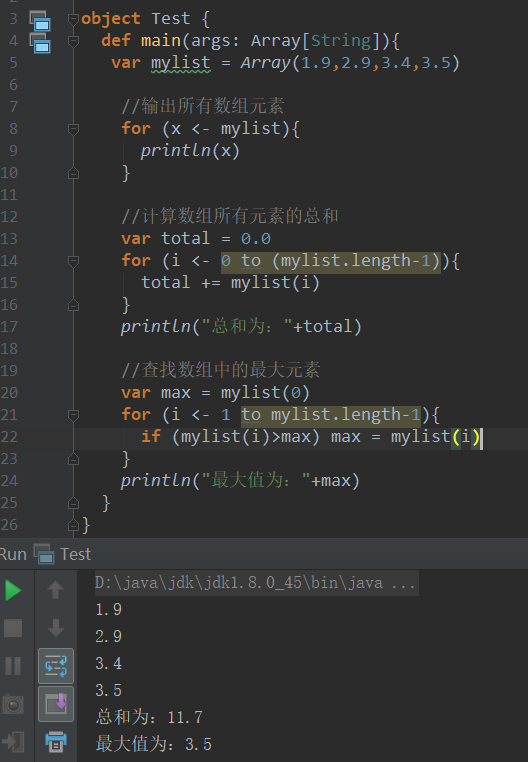### 多维数组

eg：二维数组实例：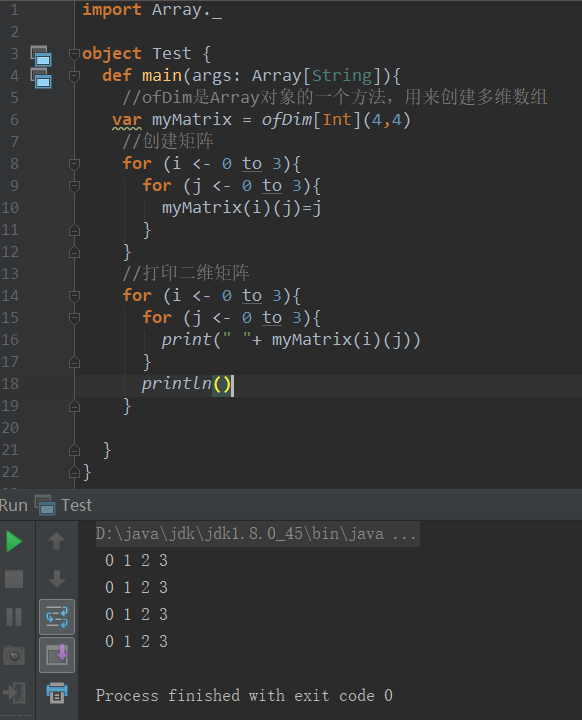### 合并数组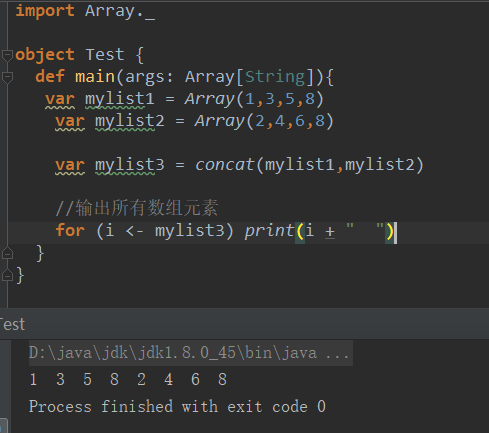### 创建区间数组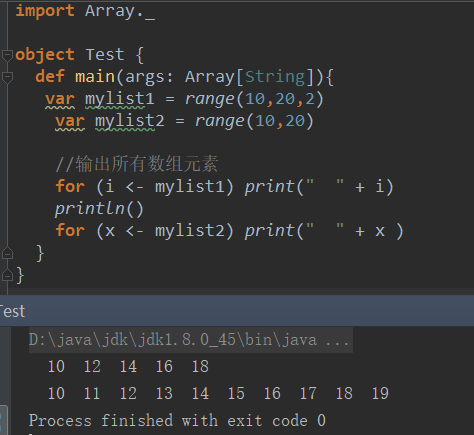### Scala 数组方法

1

def apply( x: T, xs: T* ): Array[T]

2

def concat[T]( xss: Array[T]* ): Array[T]

3

def copy( src: AnyRef, srcPos: Int, dest: AnyRef, destPos: Int, length: Int ): Unit

4

def empty[T]: Array[T]

5

def iterate[T]( start: T, len: Int )( f: (T) => T ): Array[T]

scala> Array.iterate(0,3)(a=>a+1)
res1: Array[Int] = Array(0, 1, 2)
6

def fill[T]( n: Int )(elem: => T): Array[T]

7

def fill[T]( n1: Int, n2: Int )( elem: => T ): Array[Array[T]]

8

def ofDim[T]( n1: Int ): Array[T]

9

def ofDim[T]( n1: Int, n2: Int ): Array[Array[T]]

10

def ofDim[T]( n1: Int, n2: Int, n3: Int ): Array[Array[Array[T]]]

11

def range( start: Int, end: Int, step: Int ): Array[Int]

12

def range( start: Int, end: Int ): Array[Int]

13

def tabulate[T]( n: Int )(f: (Int)=> T): Array[T]

scala> Array.tabulate(3)(a => a + 5)
res0: Array[Int] = Array(5, 6, 7)
14

def tabulate[T]( n1: Int, n2: Int )( f: (Int, Int ) => T): Array[Array[T]]

### 二、Scala Collection

Scala提供了一套很好的集合实现，提供了一些集合类型的抽象。

Scala 集合分为可变的和不可变的集合。

• 可变集合可以在适当的地方被更新或扩展。意味着可以修改，添加，移除一个集合的元素。
• 而不可变集合类，相比之下，永远不会改变。不过，你仍然可以模拟添加，移除或更新操作。但是这些操作将在每一种情况下都返回一个新的集合，同时使原来的集合不发生改变。

eg：集合类型的定义实例↓

//定义整型List
val x = List(1,2,3,4)
//定义Set
val y = Set(1,3,5,7)
//定义map
var z = Map("one"->1,"two"->2,"three"->3)
//创建两个不同类型元素的元组
val a = (10,"Runnob")

//定义option
val b:Option[Int] = Some(5)

### 1、List（列表）

List的特征是其元素以线性方式存储，集合中可以存放重复对象。

• Scala 列表类似于数组，所有元素的类型都相同，不同在于：列表是不可变的，值一旦被定义了就不能改变，其次列表 具有递归的结构（也就是链接表结构）而数组不是。。

eg：

//字符串列表
//整型列表
val nums:List[Int] = List(1,2,3,4)
//空列表
val empty:List[Nothing] = List()
//二维列表
val dim:List[List[Int]] =
List(
List(1,0,0),
List(0,1,0),
List(0,0,1)
)
• 构造列表的两个基本单位是 Nil::
• Nil 也可以表示为一个空列表。

//字符串列表
val site = "Runnob" :: ("Google" :: ("Baidu" :: Nil))
//整型列表
val nums:List[Int] = List(1,2,3,4)
val num = 1 :: (2 :: (3 ::(4 :: Nil)))
//空列表
val empty = Nil
//二维列表
val dim =(1 :: (0 :: (0 ::Nil))) ::
(0 :: (1 :: (0 ::Nil))) ::
(0 :: (0 :: (1 ::Nil))) ::Nil

### 列表基本操作

Scala列表有三个基本操作：

• head 返回列表第一个元素
• tail 返回一个列表，包含除了第一元素之外的其他元素
• isEmpty 在列表为空时返回true

val site = "Runnob" :: ("Google" :: ("Baidu" :: Nil))
val nums = Nil
println("最后一个网站是：" + site.tail)
println("查看列表site是否为空：" + site.isEmpty)
println("查看buns是否为空：" + nums.isEmpty)

### 连接列表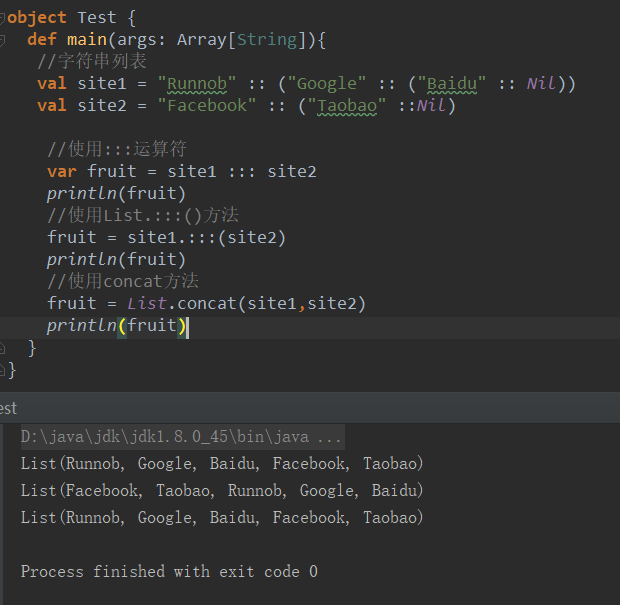### List.fill()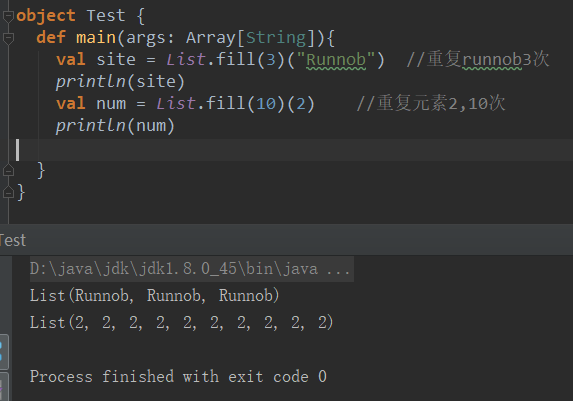## List.tabulate()

List.tabulate() 方法是通过给定的函数来创建列表。

• 方法的第一个参数为元素的数量，可以是二维的，第二个参数为指定的函数
• 通过指定的函数计算结果并返回值插入到列表中，起始值为 0
• eg：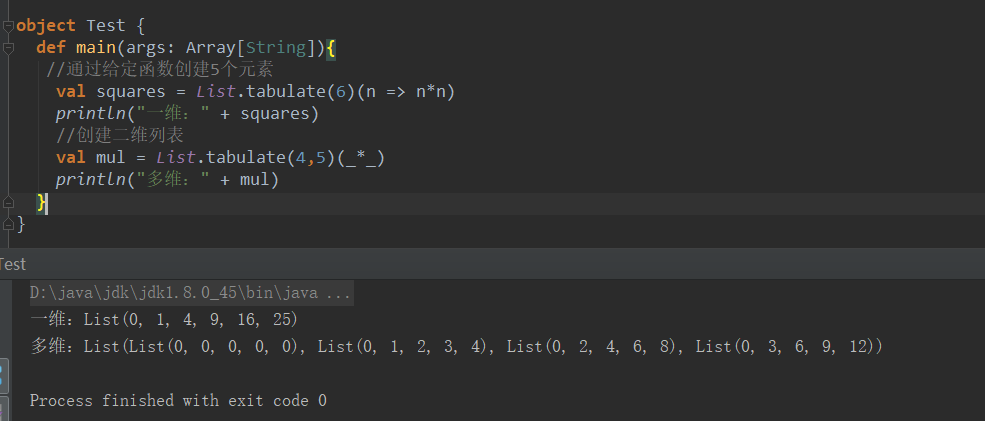### List.reverse

List.reverse 用于将列表的顺序反转，eg：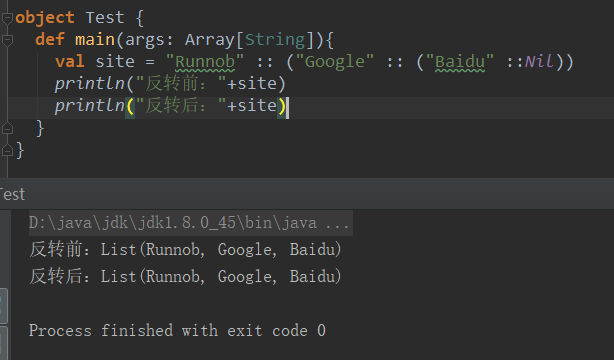### Scala List 常用方法

1

def +:(elem: A): List[A]

scala> val x = List(1)
x: List[Int] = List(1)

scala> val y = 2 +: x
y: List[Int] = List(2, 1)

scala> println(x)
List(1)
2

def ::(x: A): List[A]

3

def :::(prefix: List[A]): List[A]

4

def :+(elem: A): List[A]

scala> val a = List(1)
a: List[Int] = List(1)

scala> val b = a :+ 2
b: List[Int] = List(1, 2)

scala> println(a)
List(1)
5

6

def addString(b: StringBuilder, sep: String): StringBuilder

7

def apply(n: Int): A

8

def contains(elem: Any): Boolean

9

def copyToArray(xs: Array[A], start: Int, len: Int): Unit

10

def distinct: List[A]

11

def drop(n: Int): List[A]

12

def dropRight(n: Int): List[A]

13

def dropWhile(p: (A) => Boolean): List[A]

14

def endsWith[B](that: Seq[B]): Boolean

15

def equals(that: Any): Boolean

16

def exists(p: (A) => Boolean): Boolean

scala> l.exists(s => s == "Hah")
res7: Boolean = true
17

def filter(p: (A) => Boolean): List[A]

scala> l.filter(s => s.length == 3)
res8: List[String] = List(Hah, WOW)
18

def forall(p: (A) => Boolean): Boolean

scala> l.forall(s => s.startsWith("H")) res10: Boolean = false
19

def foreach(f: (A) => Unit): Unit

20

21

def indexOf(elem: A, from: Int): Int

22

def init: List[A]

23

def intersect(that: Seq[A]): List[A]

24

def isEmpty: Boolean

25

def iterator: Iterator[A]

26

def last: A

27

def lastIndexOf(elem: A, end: Int): Int

28

def length: Int

29

def map[B](f: (A) => B): List[B]

30

def max: A

31

def min: A

32

def mkString: String

33

def mkString(sep: String): String

34

def reverse: List[A]

35

def sorted[B >: A]: List[A]

36

def startsWith[B](that: Seq[B], offset: Int): Boolean

37

def sum: A

38

def tail: List[A]

39

def take(n: Int): List[A]

40

def takeRight(n: Int): List[A]

41

def toArray: Array[A]

42

def toBuffer[B >: A]: Buffer[B]

43

def toMap[T, U]: Map[T, U]

List 转换为 Map

44

def toSeq: Seq[A]

List 转换为 Seq

45

def toSet[B >: A]: Set[B]

List 转换为 Set

46

def toString(): String

### 2、Set（集合）

Set是最简单的一种集合。集合中的对象不按特定的方式排序，并且没有重复对象。

• Scala Set(集合)是没有重复的对象集合，所有的元素都是唯一的。

Scala 集合分为可变的和不可变的集合。

• 默认情况下，Scala 使用的是不可变集合，若想使用可变集合，需要引用 scala.collection.mutable.Set 包。
• 默认引用 scala.collection.immutable.Set
• eg: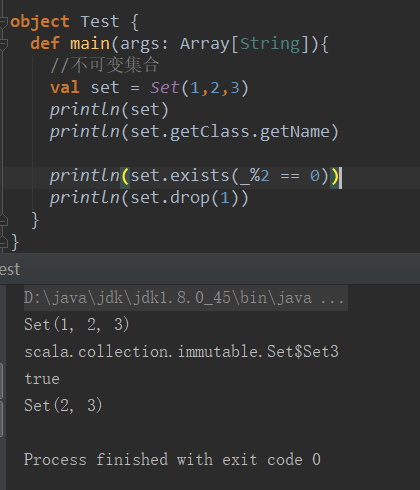eg：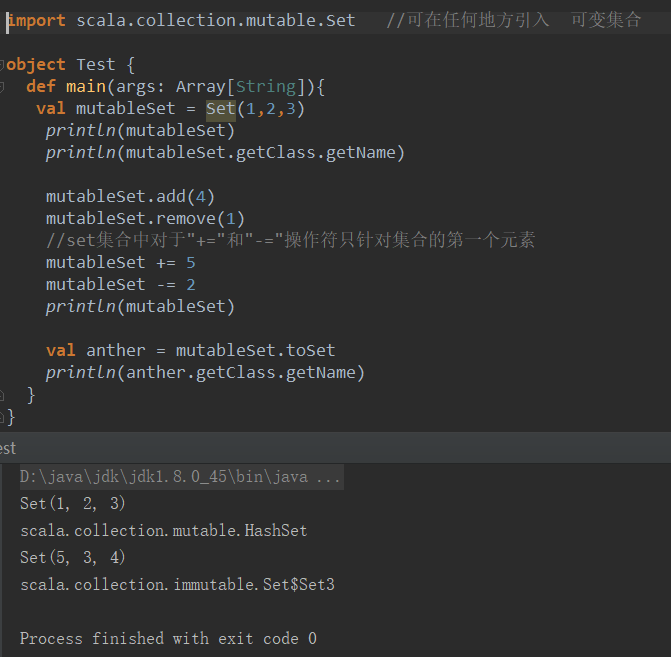• 对不可变Set进行操作，会产生一个新的set，原来的set并没有改变，这与List一样。
• 而对可变Set进行操作，改变的是该Set本身，与ListBuffer类似。

### 集合基本操作

Scala集合有三个基本操作：

eg：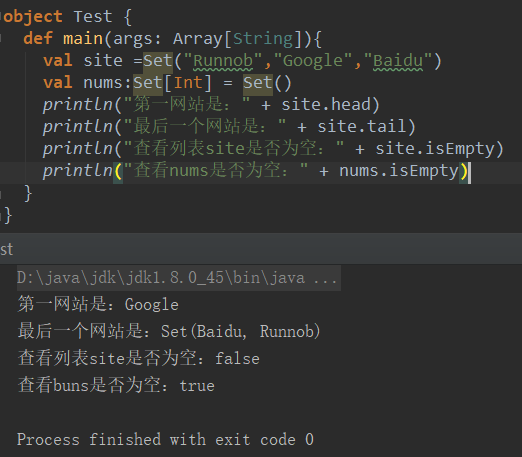• head 返回集合第一个元素
• tail 返回一个集合，包含除了第一元素之外的其他元素
• isEmpty 在集合为空时返回true

### 连接集合

eg：

object Test {
def main(args: Array[String]){

//++作为运算符使用
var site = site1 ++ site2

//使用++作为方法使用
site = site1.++(site2)
}
}

### 查找集合中最大与最小元素

eg：

object Test {
def main(args: Array[String]){
val num = Set(4,7,9,25,7,13,75)
//查找集合中的最值元素
println("max:" + num.max)     //max:75
println("min:" + num.min)     //min:4
}
}

### 交集

object Test {
def main(args: Array[String]){
val num1 = Set(4,7,9,25,7,13,75)
val num2 = Set(7,25,45,13,54,6,3)

//交集
println("Set.&:" + num1.&(num2))       //Set.&:Set(13, 7, 25)
println("Set.intersect:" + num1.intersect(num2))     //Set.intersect:Set(13, 7, 25)
}
}

### Scala Set 常用方法

1

def +(elem: A): Set[A]

2

def -(elem: A): Set[A]

3

def contains(elem: A): Boolean

4

def &(that: Set[A]): Set[A]

5

def &~(that: Set[A]): Set[A]

6

def +(elem1: A, elem2: A, elems: A*): Set[A]

7

def ++(elems: A): Set[A]

8

def -(elem1: A, elem2: A, elems: A*): Set[A]

9

10

def addString(b: StringBuilder, sep: String): StringBuilder

11

def apply(elem: A)

12

def count(p: (A) => Boolean): Int

13

def copyToArray(xs: Array[A], start: Int, len: Int): Unit

14

def diff(that: Set[A]): Set[A]

15

def drop(n: Int): Set[A]]

16

def dropRight(n: Int): Set[A]

17

def dropWhile(p: (A) => Boolean): Set[A]

18

def equals(that: Any): Boolean

equals 方法可用于任意序列。用于比较系列是否相等。

19

def exists(p: (A) => Boolean): Boolean

20

def filter(p: (A) => Boolean): Set[A]

21

def find(p: (A) => Boolean): Option[A]

22

def forall(p: (A) => Boolean): Boolean

23

def foreach(f: (A) => Unit): Unit

24

25

def init: Set[A]

26

def intersect(that: Set[A]): Set[A]

27

def isEmpty: Boolean

28

def iterator: Iterator[A]

29

def last: A

30

def map[B](f: (A) => B): immutable.Set[B]

31

def max: A

32

def min: A

33

def mkString: String

34

def mkString(sep: String): String

35

def product: A

36

def size: Int

37

def splitAt(n: Int): (Set[A], Set[A])

38

def subsetOf(that: Set[A]): Boolean

39

def sum: A

40

def tail: Set[A]

41

def take(n: Int): Set[A]

42

def takeRight(n: Int):Set[A]

43

def toArray: Array[A]

44

def toBuffer[B >: A]: Buffer[B]

45

def toList: List[A]

46

def toMap[T, U]: Map[T, U]

47

def toSeq: Seq[A]

48

def toString(): String

### 3、Map（映射）

Map ：键值对映射的结合，每一个元素都包括一个key和一个value

• Map(映射)是一种可迭代的键值对（key/value）结构。
• 所有的值都可以通过键来获取。
• Map 中的键都是唯一的。
• Map 也叫哈希表（Hash tables）

Map 有两种类型，可变与不可变，区别在于可变对象可以修改它，而不可变对象不可以。

• 默认情况下 Scala 使用不可变 Map。如果需要使用可变集合，需要显式的引入 import scala.collection.mutable.Map
• 在 Scala 中 可以同时使用可变与不可变 Map，不可变的直接使用 Map，可变的使用 mutable.Map。

eg：

//不可变map
//空哈希表，键为字符串，值为整型
var A:Map[Char,Int] = Map()
//键值对演示
val colors = Map("red" -> "#FF0000","azure" -> "#F0FFFF")
• 定义 Map 时，需要为键值对定义类型。如果需要添加 key-value 对，可以使用 +
   eg：A += ('I' -> 1)   此处注意I用的是单引号

### Map 基本操作

Scala Map 有三个基本操作：

keys返回 Map 所有的键(key)
values返回 Map 所有的值(value)
isEmpty在 Map 为空时返回true

eg：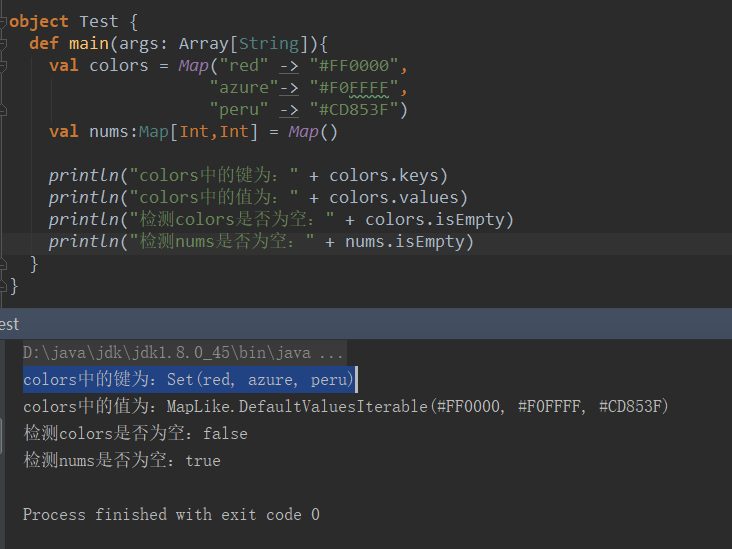### Map 合并

eg：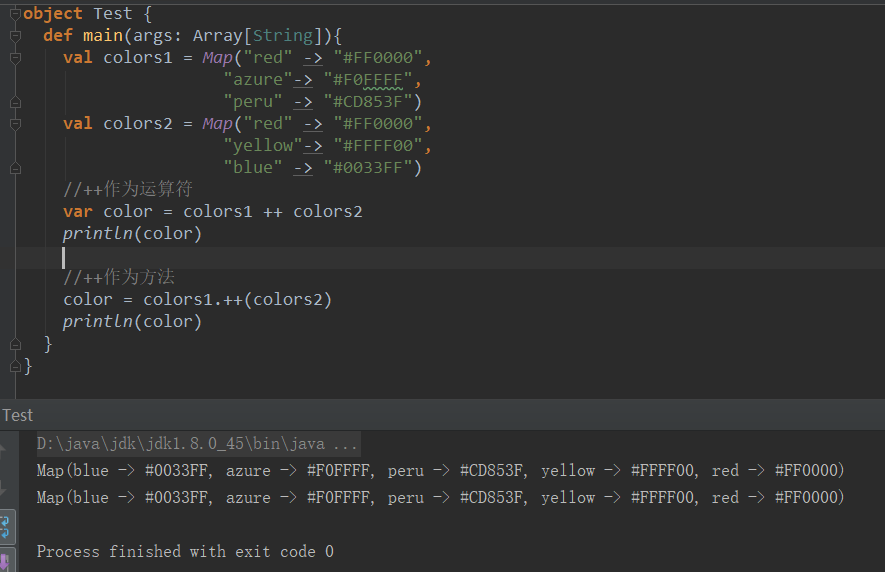### 输出 Map 的 keys 和 values

eg：通过 foreach 循环输出 Map 中的 keys 和 values：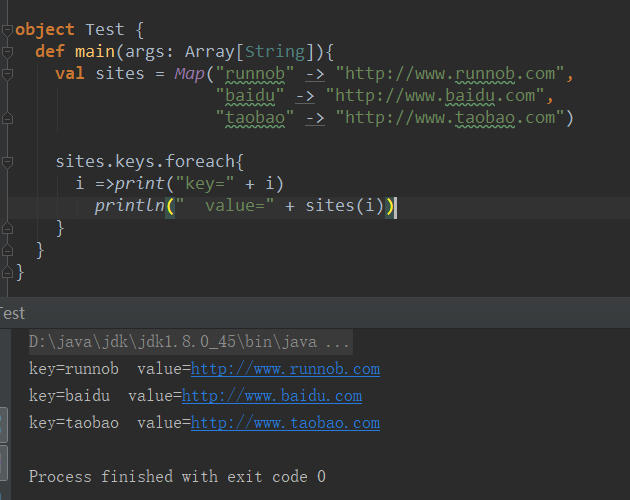### 查看 Map 中是否存在指定的 Key

eg：

object Test {
def main(args: Array[String]){
val sites = Map("runnob" -> "http://www.runnob.com",
"baidu" -> "http://www.baidu.com",
"taobao" -> "http://www.taobao.com")

if (sites.contains("runnob")){
println("runnob键存在，对应的值为：" + sites("runnob"))
}else{
println("runnob键不存在！")
}
}
}

### Scala Map 方法

1

def ++(xs: Map[(A, B)]): Map[A, B]

2

def -(elem1: A, elem2: A, elems: A*): Map[A, B]

3

def --(xs: GTO[A]): Map[A, B]

4

def get(key: A): Option[B]

5

def iterator: Iterator[(A, B)]

6

7

def addString(b: StringBuilder, sep: String): StringBuilder

8

def apply(key: A): B

9

def clear(): Unit

10

def clone(): Map[A, B]

11

def contains(key: A): Boolean

12

def copyToArray(xs: Array[(A, B)]): Unit

13

def count(p: ((A, B)) => Boolean): Int

14

def default(key: A): B

15

def drop(n: Int): Map[A, B]

16

def dropRight(n: Int): Map[A, B]

17

def dropWhile(p: ((A, B)) => Boolean): Map[A, B]

18

def empty: Map[A, B]

19

def equals(that: Any): Boolean

20

def exists(p: ((A, B)) => Boolean): Boolean

21

def filter(p: ((A, B))=> Boolean): Map[A, B]

22

def filterKeys(p: (A) => Boolean): Map[A, B]

23

def find(p: ((A, B)) => Boolean): Option[(A, B)]

24

def foreach(f: ((A, B)) => Unit): Unit

25

def init: Map[A, B]

26

def isEmpty: Boolean

27

def keys: Iterable[A]

28

def last: (A, B)

29

def max: (A, B)

30

def min: (A, B)

31

def mkString: String

32

def product: (A, B)

33

def remove(key: A): Option[B]

34

def retain(p: (A, B) => Boolean): Map.this.type

35

def size: Int

36

def sum: (A, B)

37

def tail: Map[A, B]

38

def take(n: Int): Map[A, B]

39

def takeRight(n: Int): Map[A, B]

40

def takeWhile(p: ((A, B)) => Boolean): Map[A, B]

41

def toArray: Array[(A, B)]

42

def toBuffer[B >: A]: Buffer[B]

43

def toList: List[A]

44

def toSeq: Seq[A]

45

def toSet: Set[A]

46

def toString(): String

### 4、元组

• 与列表一样，元组也是不可变的，但与列表不同的是元组可以包含不同类型的元素。
• 元组的值是通过将单个的值包含在圆括号中构成的。

eg：

val a = (1,3.14,"Fred")
val b = new Tuple3(1,3.14,"Fred")
• 元组的实际类型取决于它的元素的类型

• 可以通过数字索引访问元组的元素

eg：可以使用 t._1 访问第一个元素， t._2 访问第二个元素

object Test {
def main(args: Array[String]){
val t = (4,3,2,1)
val sum = t._1+t._2+t._3+t._4
println("元素之和：" + sum)    //元素之和：10

}
}

### 迭代元组

eg：

object Test {
def main(args: Array[String]){
val t = (4,3,2,1)
t.productIterator.foreach{i => println("value = " + i)}
/*运行结果：
value = 4
value = 3
value = 2
value = 1
*/
}
}

### 元组转为字符串

eg：

object Test {
def main(args: Array[String]){
val t = new Tuple3(1,"hello",Console)
println("连接后的字符串：" + t.toString())   //连接后的字符串：(1,hello,scala.Console\$@256216b3)
}
}

### 元素交换

eg：

object Test {
def main(args: Array[String]){
}
}

### 5、Option

Option[T] 表示有可能包含值的容器，也可能不包含值。

• Scala Option(选项)类型用来表示一个值是可选的（有值或无值)。
• Option[T] 是一个类型为 T 的可选值的容器： 如果值存在， Option[T] 就是一个 Some[T] ，如果不存在， Option[T] 就是对象 None 。

eg：

object Test {
def main(args: Array[String]){
val myMap:Map[String,String] = Map("key1" -> "value")
val value1:Option[String] = myMap.get("key1")
val value2:Option[String] = myMap.get("key2")

println(value1)   //Some(value)
println(value2)   //None
}
}

eg：

• Scala 使用 Option[String] 来告诉你：「我会想办法回传一个 String，但也可能没有 String 给你」。
• myMap 里并没有 key2 这笔数据，get() 方法返回 None。
• Option 有两个子类别，一个是 Some，一个是 None，当他回传 Some 的时候，代表这个函式成功地给了你一个 String，而你可以透过 get() 这个函式拿到那个 String，如果他返回的是 None，则代表没有字符串可以给你。
val sites = Map("runnob" -> "www.runnob.com","google" -> "www.google.com")
println(sites.get("runnob"))  //Some(www.runnob.com)
println(sites.get("baidu"))    //None

eg：

object Test {
def show(x:Option[String])= x match {
case Some(s) => s
case None => "?"
}
def main(args: Array[String]){
println(show(sites.get("runnob")))  //www.runnob.com
println(show(sites.get("baidu")))    //?
}
}

### getOrElse() 方法

object Test {
def main(args: Array[String]){
val a:Option[Int] = Some(5)
val b:Option[Int] = None

println(a.getOrElse(0))      //5
println(b.getOrElse(10))     //10
}
}

### isEmpty() 方法

object Test {
def main(args: Array[String]){
val a:Option[Int] = Some(5)
val b:Option[Int] = None

println(a.isEmpty)      //false
println(b.isEmpty)     //true
}
}

### Scala Option 常用方法

1

def get: A

2

def isEmpty: Boolean

3

def productArity: Int

4

def productElement(n: Int): Any

5

def exists(p: (A) => Boolean): Boolean

6

def filter(p: (A) => Boolean): Option[A]

7

def filterNot(p: (A) => Boolean): Option[A]

8

def flatMap[B](f: (A) => Option[B]): Option[B]

9

def foreach[U](f: (A) => U): Unit

10

def getOrElse[B >: A](default: => B): B

11

def isDefined: Boolean

12

def iterator: Iterator[A]

13

def map[B](f: (A) => B): Option[B]

14

def orElse[B >: A](alternative: => Option[B]): Option[B]

15

def orNull

### 三、Iterator（迭代器）

• Scala Iterator（迭代器）不是一个集合，它是一种用于访问集合的方法。
• 迭代器 it 的两个基本操作是 nexthasNext
• 调用 it.next() 会返回迭代器的下一个元素，并且更新迭代器的状态。
• 调用 it.hasNext() 用于检测集合中是否还有元素。

eg：

object Test {
def main(args: Array[String]){
while (it.hasNext){
println(it.next())
}
}
}

运行结果：

Baidu
Runnob
Taobao

### 查找最大与最小元素

eg：

object Test {
def main(args: Array[String]){
var ita = Iterator(20,40,3,20,6,30)
var itb = Iterator(20,40,3,20,6,30)
println("max:" + ita.max)
println("min:" + itb.min)
}
}


### 获取迭代器的长度

eg：

object Test {
def main(args: Array[String]){
var ita = Iterator(20,40,3,20,6,30)
var itb = Iterator(20,40,3,20,6,30)
println("size:" + ita.size)      //size:6
println("length:" + itb.length)   // length:6
}
}

### Scala Iterator 常用方法

1

def hasNext: Boolean

2

def next(): A

3

def ++(that: => Iterator[A]): Iterator[A]

4

def ++[B >: A](that :=> GenTraversableOnce[B]): Iterator[B]

5

6

def addString(b: StringBuilder, sep: String): StringBuilder

7

def buffered: BufferedIterator[A]

8

def contains(elem: Any): Boolean

9

def copyToArray(xs: Array[A], start: Int, len: Int): Unit

10

def count(p: (A) => Boolean): Int

11

def drop(n: Int): Iterator[A]

12

def dropWhile(p: (A) => Boolean): Iterator[A]

13

def duplicate: (Iterator[A], Iterator[A])

14

def exists(p: (A) => Boolean): Boolean

15

def filter(p: (A) => Boolean): Iterator[A]

16

def filterNot(p: (A) => Boolean): Iterator[A]

17

def find(p: (A) => Boolean): Option[A]

18

def flatMap[B](f: (A) => GenTraversableOnce[B]): Iterator[B]

19

def forall(p: (A) => Boolean): Boolean

20

def foreach(f: (A) => Unit): Unit

21

def hasDefiniteSize: Boolean

22

def indexOf(elem: B): Int

23

def indexWhere(p: (A) => Boolean): Int

24

def isEmpty: Boolean

25

def isTraversableAgain: Boolean

Tests whether this Iterator can be repeatedly traversed.

26

def length: Int

27

def map[B](f: (A) => B): Iterator[B]

28

def max: A

29

def min: A

30

def mkString: String

31

def mkString(sep: String): String

32

def nonEmpty: Boolean

33

def padTo(len: Int, elem: A): Iterator[A]

34

def patch(from: Int, patchElems: Iterator[B], replaced: Int): Iterator[B]

35

def product: A

36

def sameElements(that: Iterator[_]): Boolean

37

def seq: Iterator[A]

38

def size: Int

39

def slice(from: Int, until: Int): Iterator[A]

40

def sum: A

41

def take(n: Int): Iterator[A]

42

def toArray: Array[A]

43

def toBuffer: Buffer[B]

44

def toIterable: Iterable[A]

Returns an Iterable containing all elements of this traversable or iterator. This will not terminate for infinite iterators.

45

def toIterator: Iterator[A]

46

def toList: List[A]

47

def toMap[T, U]: Map[T, U]

48

def toSeq: Seq[A]

49

def toString(): String

50

def zip[B](that: Iterator[B]): Iterator[(A, B)

08-147106
02-1721304-29740
08-037156
06-075127
10-232338
09-21276
02-094397
01-125909
02-075099
04-1659
09-21189
03-034万+
07-243996
10-251557
12-016万+
10-233447
08-012720
06-182347点击重新获取扫码支付余额充值